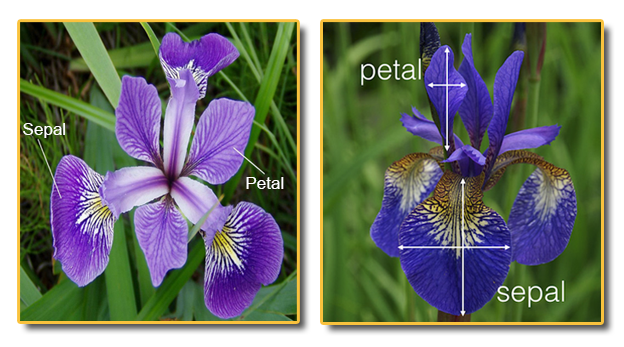# Introduction¶

## First data science example: species delimitation¶

Course: Math 535 - Mathematical Methods in Data Science (MMiDS)
Author: Sebastien Roch, Department of Mathematics, University of Wisconsin-Madison
Updated: August 28, 2020

Imagine that you are an evolutionary biologist studying irises and that you have collected measurements on a large number of iris samples. Your goal is to identify different species within this collection.(Source)

Here is a classical iris dataset first analyzed by Fisher. We will upload the data in the form of a DataFrame -- similar to a spreadsheet -- where the columns are different measurements (or features) and the rows are different samples. Below, we show the first $5$ lines of the dataset.

In :
# Julia version: 1.5.1
using CSV, DataFrames, Statistics, Plots, LinearAlgebra, StatsPlots

In :
df = CSV.read("iris-measurements.csv")
first(df, 5)

Out:

5 rows × 5 columns

IdPetalLengthCmPetalWidthCmSepalLengthCmSepalWidthCm
Int64Float64Float64Float64Float64
111.40.25.13.5
221.40.24.93.0
331.30.24.73.2
441.50.24.63.1
551.40.25.03.6

There are $150$ samples.

In :
nrow(df)

Out:
150

Here is a summary of the data.

In :
describe(df)

Out:

5 rows × 8 columns

variablemeanminmedianmaxnuniquenmissingeltype
SymbolFloat64RealFloat64RealNothingNothingDataType
1Id75.5175.5150Int64
2PetalLengthCm3.758671.04.356.9Float64
3PetalWidthCm1.198670.11.32.5Float64
4SepalLengthCm5.843334.35.87.9Float64
5SepalWidthCm3.0542.03.04.4Float64

Let's first extract the columns into vectors and combine them into a matrix, and visualize the petal data. Below, each point is a sample. This is called a scatter plot.

In :
X = reduce(hcat,
[df[:,:PetalLengthCm], df[:,:PetalWidthCm],
df[:,:SepalLengthCm], df[:,:SepalWidthCm]]);

In :
scatter(X[:,1], X[:,2],
legend=false, xlabel="PetalLength", ylabel="PetalWidth")

Out:

Observe a clear cluster of samples on the bottom left. This may be an indication that these samples come from a separate species. What is a cluster? Intuitively, it is a group of samples that are close to each other, but far from every other sample.

Now let's look at the full dataset. Visualizing the full $4$-dimensional data is not so straighforward. One way to do this is to consider all pairwise scatter plots.

In :
cornerplot(X,
label=["PetalWidth", "PetalLength", "SepalWidth", "SepalLength"],
size=(500,500),
markersize=2)

Out:

We would like a method that automatically identifies clusters -- whatever the dimension of the data. We will discuss a standard way to do this: $k$-means clustering.

This topic has two main goals:

1. To review basic facts about Euclidean geometry, vector calculus, probability, and matrix algebra.
2. To introduce a first data science problem and highlight some relevant, surprising phenomena arising in high-dimensional space.

We will come back to the iris dataset in an accompanying tutorial notebook.